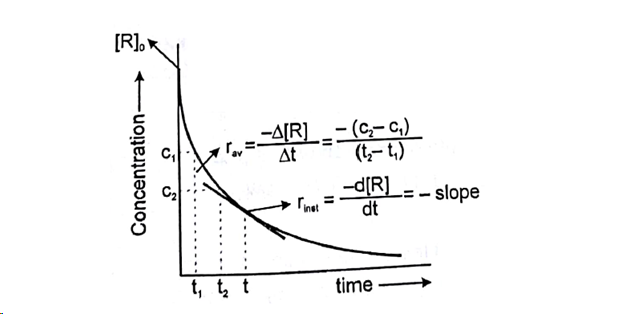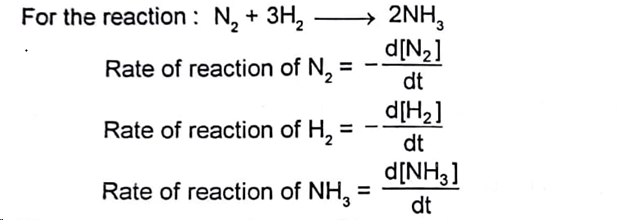# Chemical Reaction: Rate/Velocity, Types of Rates, Relation between Reaction Rates

Rate/Velocity of chemical reaction

The rate of change of concentration with time of different chemical species taking part in a chemical reaction is known as rate of reaction of that species.Rate is always defined in such a manner so that it is always a positive quantity.

Types of Rates of chemical reaction

For a reaction R à P

Average rate = Total change in concentration / Total time Taken

Instantaneous Rate: Rate of reaction at a particular instant.

Instantaneous rate can be determined by drawing a tangent at time t on curve drawn for concentration versus time.

Initial Rate: Instantaneous rate ‘t = 0’ is called initial rate [slope of tangent at t = 0]Relation between reaction rates of different species involved is a reactionThese rates are not equal. Therefore, by convention the rate of a reaction is defined as

Note: Rate of reaction value is dependent on the stoichiometric coefficients used in the reaction while rate of any species will be fixed value under given conditions.

Post By : Mona Thakur 21 Feb, 2019 1980 views Chemistry